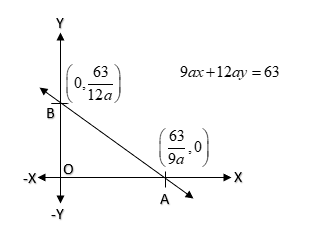QUESTION

# If x = 1, y = 1 is a solution of the equation $9ax + 12ay = 63$ Then, what is the value of a?A.-3B.3C.7D.5

Hint- We approach this problem in the way that we will substitute the values of x and y given in the equation as they are the solutions of the equation so they will satisfy the equation. After that the equation will be left out with the one variable which we can easily calculate out using basic arithmetic operations of equality.Equation so given is $9ax + 12ay = 63$
Solutions of the equation so given are x = 1, y = 1
On substituting the values of x and y in the equation we get
$\Rightarrow 9a \times 1 + 12a \times 1 = 63$
$\Rightarrow 9a + 12a = 63$
$\Rightarrow 21a = 63$
$\Rightarrow a = \dfrac{{63}}{{21}}$
$\Rightarrow a = 3$
Hence, the required value of a is 3
$\therefore$ Option B. 3 is our correct answer.

Note- For such types of questions, just keep in mind that we have to substitute the solution in the linear equation due to which we get left out with the variable whose value we want to find out. Solution of a linear equation is the set of points (x, y) which when we substitute into the equation the equation gets satisfied.